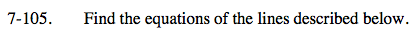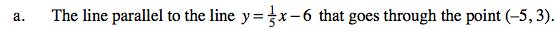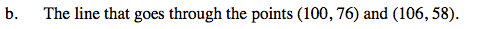### Home > AC > Chapter 7 > Lesson 7.3.3 > Problem7-105

7-105.Because the lines are parallel, they have the same slope.
Therefore, substitute the given (x, y) coordinate into the equation for a line with the same slope as the equation above.

$y=\frac{1}{5}x-6 \rightarrow 3=\frac{1}{5}(-5)+b$

Now solve for b and rewrite the equation with x and y substituted back into their original positions.

$y=\frac{1}{5}x+4$Make a slope triangle and find the differences in x values and y values to find the length of the legs of the triangle.
Use the values to find the slope.

Substitute one of the coordinates into the equation of a line, where m = −3, to solve for b.

Rewrite the equation with x and y substituted back into their original positions.